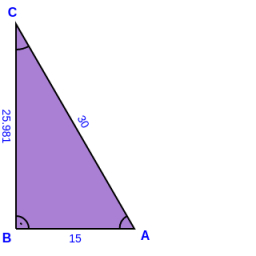# Cosine

Cosine and sine theorem:
Calculate all missing values (sides and angles) of the triangle ABC.

a = 20 cm; b = 15 cm; γ = 90°; c =? cm; α =? °; β =? °

c =  25 cm
α =  53.1301 °
β =  36.8699 °

### Step-by-step explanation:

Try calculation via our triangle calculator.Did you find an error or inaccuracy? Feel free to write us. Thank you!

Tips to related online calculators
Need help to calculate sum, simplify or multiply fractions? Try our fraction calculator.